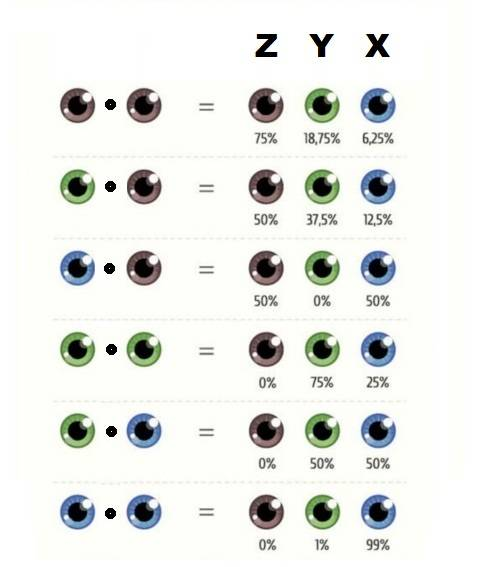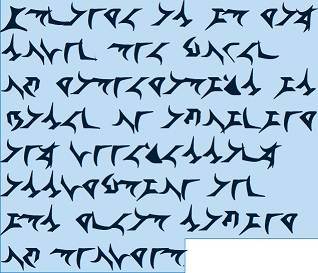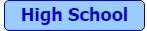# Math Challenge - March 2019

• Challenge
• Featured
Mentor
Questions

1.)
(disclosed by @Demystifier ) Using the notion of double integrals prove that $$B(m,n) = \frac{\Gamma (m) \Gamma (n)}{\Gamma (m + n)}\; \;(m \gt 0\,,\, n\gt 0)$$ where ##B## and ##\Gamma## are the Beta and Gamma functions respectively.

2.) (solved by @Math_QED ) Show that the Fourier series of an even function cannot contain sine.

3.) (solved by @Bosko ) Show that $$\int_{0}^{1} dx \int_{x}^{\sqrt{x}} f(x,y)\,dy = \int_{0}^{1} dy \int_{y^2}^{y} f(x,y)\,dx\,.$$
4.) The graphic of eye colors shows us the probability of baby's eye color in dependency of the parents'. This yields the following multiplication table:\begin{align*}
x \cdot x &= (3/4) x + (3/16) y + (1/16) z\\
x \cdot y &=(1/2) x+(3/8) y+(1/8) z\\
x \cdot z &=(1/2) x+(1/2) z\\
y \cdot y &=(3/4) y+(1/4) z\\
y \cdot z &=(1/2) y+(1/2) z\\
z \cdot z &= z
\end{align*}
which we extend to a real, commutative, distributive, three dimensional algebra ##A##.

a) (solved by @fbs7 ) Is ##A## an associative algebra?

still open:

b) Prove, that ##A## is a baric algebra, i.e. show that there is a non trivial algebra homomorphismus ##\omega\, : \,A\longrightarrow \mathbb{R}##, the weight function.
c) Determine a basis for ##\operatorname{ker}\omega## and rewrite the multiplication table according to this new basis.
d) Prove that there is an ideal ##N## of codimension one in ##A##, such that ##A^2 \nsubseteq N##.
e) A algebra is called genetic, if there is a basis ##\{\,u_i\,\}## such that the structure constants ##\lambda_{ijk}## defined by
$$u_i \cdot u_j = \sum_{k=1}^n \lambda_{ijk}u_k$$
fulfill the following conditions:
• ##\lambda_{111}=1##
• ##\lambda_{1jk}=0## for all ##j>k##
• ##\lambda_{ijk}=0## for all ##i,j > 1## and ##k \leq \operatorname{max}\{i,j\}##
Prove that all genetic algebras are baric algebras.
f) Show that ##A## is not a genetic algebra.
g) Determine all idempotent elements of ##A##. Is there a basis of ##A## with idempotent elements?

5.) (solved by @Bosko ) Prove that starting with ##\frac{1}{1}## the following binary tree
$$\begin{array}{ccccc} &&\frac{a}{b}&&\\ &\swarrow &&\searrow \\ \frac{a}{a+b}&&&&\frac{a+b}{b} \end{array}$$
defines a counting of all positive rational numbers without repetition and all quotients canceled out.

6.) (solved by @Cthugha ) Who said what here? (What is the task, who a bonus.)7.) (solved by @lpetrich ) Calculate ##I:=\int_0^\infty \dfrac{\sqrt{x^{e-2}}}{x^e+1}\,dx##

8.) An algebra ##A## is a vector space with a binary distributive multiplication. An example are group algebras, i.e. the distributive extension of the formal basis vectors ##g\in G## such as $$A :=\mathbb{R}[S_3]=\mathbb{R}\cdot(1)+\mathbb{R}\cdot(12)+\mathbb{R}\cdot(13)+\mathbb{R}\cdot(23)+\mathbb{R}\cdot(123)+\mathbb{R}\cdot(132)$$
a.) Find the center ##Z(A)=\{\,z \in A\,|\,zv=vz\text{ for all }v\in A\,\}## of ##A##, and
b.) determine the structure of ##A##, i.e. its decomposition into direct factors and the corresponding isomorphisms.

9.) Let ##B\subseteq \mathbb{R}^n## be measurable and ##P=(a_1,\ldots,a_n,b)\in \mathbb{R}^{n+1}## a point with ##b>0## and ##C_B=\{\,P+t(Q-P)\,|\,Q\in B\times \{0\}_{n+1}\, , \,t\in [0,1]\,\}## the cone above the basis ##B## with the peak ##P\,.##
Prove the measure formula
$$\lambda^{n+1}(C_B)=\dfrac{b}{n+1}\cdot \lambda^n(B)$$
10.) Show that $$x \cdot y = \frac{2xy-x-y}{xy-1}$$
defines a one dimensional, real, local Lie group ##G## around ##0 \in \mathbb{R}## and compute the vector field of left multiplication by an element ##g \in \mathbb{R}##.1.) (solved by @fbs7 ) Find the limit of the sequence ##a_n = 2\cdot \sqrt{4}\cdot \sqrt{16}\cdot \sqrt{256} \,\cdots \, \sqrt[3^{n-1}]{2^{2^{n-1}}}##.

2.) Let ##(b_n)## be a sequence with ##b_n = \dfrac{nn^n}{(n!)^2}##.
a.) Show that ##(b_n)## converges and find its limit.
b.) Using the above find the limit of the sequence ##(a_n)## with ##a_n = \dfrac{1^n + 2^n + 3^n + \dots + n^n}{(n!)^2}##.

3.) (solved by @fbs7 ) Two numbers ##a,b## are called amicable, if the sum of all proper divisors of one is the other number (##1## is included). The smallest example is
$$(a,b) = (220,284)=(1+2+4+71+142,1+2+4+5+10+11+20+22+44+55+110)$$
Let ##n\in \mathbb{N}## and ##(x,y,z)=\left(3\cdot 2^n-1\, , \,3\cdot 2^{n-1}-1\, , \,9\cdot 2^{2n-1}-1\right)\,.##

Prove that if ##x,y,z## are all odd primes, then ##(a,b)=\left( 2^n\cdot x\cdot y\,,\, 2^n\cdot z \right)## are amicable numbers.

Hint: First find a formula for the sum of all divisors ##\sigma(n)## given the prime decomposition of ##n##.

4.) (@fbs7 ) A number is called perfect, if it equals the number of all its divisors except itself, e.g. ##6=1+2+3## and ##28=1+2+4+7+14## are perfect.
Prove: If ##2^k-1## is a prime number, then ##2^{k-1}(2^k-1)## is a perfect number and every even perfect number has this form.

5.) a.) (solved by @fbs7 ) What is the smallest five-digit number ##n## such that ##n## and ##2n## together contain all ##10## digits from ##0## to ##9##?
b.) (solved by @fbs7 ) On how many zeros does the number ##1000\,!## end?
c.) (solved by @fbs7 ) For which six-digit number ##ABCDEF## do we have:
##ABCDEF \cdot 1 = ABCDEF##
##ABCDEF \cdot 3 = BCDEFA##
##ABCDEF \cdot 2 = CDEFAB##
##ABCDEF \cdot 6 = DEFABC##
##ABCDEF \cdot 4 = EFABCD##
##ABCDEF \cdot 5 = FABCDE##

Last edited:
•fbs7 and Greg Bernhardt

member 587159
(2)
The fourier coefficient of sine is given by (assumping period ##2\pi)##

$$a_n = 1/\pi \int_{-\pi}^{\pi} f(x) \sin(nx) dx$$

The integrand is odd, and we integrate over a symmetric interval, hence ##a_n = 0## and the result follows.

•pbuk
I like (Q5), it is a nice question.
Edit: The algorithm I thought of is quite different from construction in question. Sorry for confusion.

Last edited:
QuantumQuest
Gold Member
(2)

Your solution is basically correct - I think that you mean ##b_n## not ##a_n##, but I would prefer to write it for a general case e.g. for an interval ##(-L,L)## and take into account that ##f(x)## is an even function.

member 587159
Your solution is basically correct - I think that you mean ##b_n## not ##a_n##, but I would prefer to write it for a general case e.g. for an interval ##(-L,L)## and take into account that ##f(x)## is an even function.

This will just change the period of the sine, the integration boundaries (which remain symmetric) and another scaling constant in front. Reasoning remains exactly the same.

QuantumQuest
Gold Member
This will just change the period of the sine, the integration boundaries (which remain symmetric) and another scaling constant in front. Reasoning remains exactly the same.

Yes, I know all these. My remark is about how the solution can be presented in a more complete way. In any case, as I said in #4 your solution is basically correct.

•member 587159
member 587159
Yes, I know all these. My remark is about how the solution can be presented in a more complete way. In any case, as I said in #4 your solution is basically correct.

I will write it out with more details tomorrow, so it becomes clearer.

•QuantumQuest
I will take on Problem 7.
We must find
$$I = \int_0^\infty \frac{\sqrt{x^{e-2}}}{x^e + 1} dx$$
The first step is to evaluate the square root:
$$I = \int_0^\infty \frac{x^{e/2}}{x^e + 1} \frac{dx}{x}$$
The term ## x^{e/2} ## suggests a change of variables: ## x = y^{2/e} ##, a change that makes it ## y ##. That change of variables has the same domain of integration, and we find
$$I = \frac{2}{e} \int_0^\infty \frac{y}{y^2+1} \frac{dy}{y} = \frac{2}{e} \int_0^\infty \frac{dy}{y^2+1} = \left. \frac{2}{e} \arctan y \right|_{y=0}^\infty = \frac{\pi}{e}$$
Thus, ## I = \frac{\pi}{e} ##.

\begin{align*}
x \cdot x &= 3/4 x + 3/16 y + 1/16 z\\
x \cdot y &=1/2 x+3/8 y+1/8 z\\
x \cdot z &=1/2 x+1/2 z\\
y \cdot y &=3/4 y+1/4 z\\
y \cdot z &=1/2 y+1/2 z\\
z \cdot z &= z
\end{align*}
Coefficients or are the variables in the denominator?

Mentor
Coefficients or are the variables in the denominator?
Coefficients, sorry. I'll correct it.

•archaic
Cthugha
My take on problem 6:

"Strange as it may sound the power of mathematics is based on avoiding any unnecessary assumption and its great saving of thought"

Basic assumption: This is a cipher, where each letter corresponds to one letter in the English alphabet (which is actually an incorrect assumption, but gets you started). Word 3, word 10 and word 18 are short words that contain almost exactly the same letters. The only sensible three words in English that achieve this are "is", "its" and "it". From there on, it gets slightly more difficult. The text is a bit short for frequency analysis, but the most common letters (etaoinshr) are still common in this text, so one may start e.g. by putting vowels in. The "as" and "the" are for example easy next guesses. From there, it is possible to guess "mathematics" and then the remaining stuff is not too hard. The difficult part is that some letters correspond to two letters inthe English alphabet. The fifth letter in the picture above represents "h" and "n". and the sixth letter represents "m" and "g". Or I am too blind to tell the symbols apart accurately, which is also quite likely. ;) I also do not have the slightest idea who might have said that.

Last edited:
Mentor
My take on problem 6:

"Strange as it may sound the power of mathematics is based on avoiding any unnecessary assumption and its great saving of thought"

Basic assumption: This is a cipher, where each letter corresponds to one letter in the English alphabet (which is actually an incorrect assumption, but gets you started). Word 3, word 10 and word 18 are short words that contain almost exactly the same letters. The only sensible three words in English that achieve this are "is", "its" and "is". From there on, it gets slightly more difficult. The text is a bit short for frequency analysis, but the most common letters (etaoinshr) are still common in this text, so one may start e.g. by putting vowels in. The "as" and "the" are for example easy next guesses. From there, it is possible to guess "mathematics" and then the remaining stuff is not too hard. The difficult part is that some letters correspond to two letters inthe English alphabet. The fifth letter in the picture above represents "h" and "n". and the sixth letter represents "m" and "g". Or I am too blind to tell the symbols apart accurately, which is also quite likely. ;) I also do not have the slightest idea who might have said that.
It was Ernst Mach.

Ibix
2020 Award
It was Ernst Mach.
Indeed. You have not experienced Mach until you have read him in the original Klingon.

•fresh_42
Bosko
Gold Member
5.) Prove that starting with ##\frac{1}{1}## the following binary tree
$$\begin{array}{ccccc} &&\frac{a}{b}&&\\ &\swarrow &&\searrow \\ \frac{a}{a+b}&&&&\frac{a+b}{b} \end{array}$$
defines a counting of all positive rational numbers without repetition and all quotients canceled out.
Let choose arbitrary rational number a/b so that Greatest Common Divisor GCD(a,b)=1
Let prove that a/b is in the tree and it appears just once.

If a>b we go up-left in the tree to (a-b)/b
If a<b we go up-right in the tree to a/(b-a)
If a=b >1 then GCD(a,b) >1 -> not possible

By repeating this procedure we will reach 1/1.
The path is unique both ways either down->up or up-> down.
Every pair (a,b) have the unique path to (1,1) .
I like (Q5), it is a nice question....
I agree . It is a nice question.
By thinking about the question i noticed similarity with an algorithm ( finding Greatest Common Divisor , of a an b, algorithm )

Code:
repeat
if a>b then a=a-b
if a<b then b=b-a
until a=b
print a

Last edited:
Mentor
Let choose arbitrary rational number a/b so that Greatest Common Divisor GCD(a,b)=1
Let prove that a/b is in the tree and it appears just once.

If a>b we go up-left in the tree to (a-b)/b
If a<b we go up-right in the tree to a/(b-a)
If a=b >1 then GCD(a,b) >1 -> not possible

By repeating this procedure we will reach 1/1.
The path is unique both ways either down->up or up-> down.
Every pair (a,b) have the unique path to (1,1) .

I agree . It is a nice question.
By thinking about the question i noticed similarity with an algorithm ( finding GDC (a,b) algorithm )
repeat
if a>b then a=a-b
if a<b then b=b-a​
until a=b
You started with a quotient which is already in cancelled form. How can you rule out there are no others? And why do we get all of them?

You shouldn't start with an assumption that is part of the claim. The clue is to find a kind of measurement which allows an ordering.

Bosko
Gold Member
3.) Show that $$\int_{0}^{1} dx \int_{x}^{\sqrt{x}} f(x,y)\,dy = \int_{0}^{1} dy \int_{y^2}^{y} f(x,y)\,dx\,.$$
How can I upload image from my computer :-)

Mentor
How can I upload image from my computer :-)
You could do it with the upload button at bottom right of the edit field, but I would appreciate if you wouldn't. Here's a guideline to write formulas on PF:
https://www.physicsforums.com/help/latexhelp/

Bosko
Gold Member
You started with a quotient which is already in cancelled form.
Yes I started from arbitrary (any) a/b ...
How can you rule out there are no others?
If is not in cancelled form repeating "the algorithm" in one moment I will get a=b > 1
And why do we get all of them?
a/b can be any ... I didn't explained all steps in details
You shouldn't start with an assumption that is part of the claim. The clue is to find a kind of measurement which allows an ordering.
Yes , you are right . I explained just an idea . I should do properly , step by step with precise assumptions.

Mentor
Yes I started from arbitrary (any) a/b ...
You did not:
Let choose arbitrary rational number a/b so that Greatest Common Divisor GCD(a,b)=1
If is not in cancelled form repeating "the algorithm" in one moment I will get a=b > 1
I have no idea how this would happen.

Bosko
Gold Member
I have no idea how this would happen.
It is all about Greatest Common Divisor algorithm
Example you want to find GDC(20,12)
20 , 12
20-12 -> 8 , 12
8, 12-8->4
8-4->4 , 4
4=4 -> STOP
Explanation is that if x divides a and b then it also divides a-b
"Just repeat subtracting the smaller from the bigger one until they are both equal."
P.S. I studied Math for Computer Sciences. This is the way how computers find GCD

Last edited:
Trying high-school 5a

Running a simple search algorithm. 1st column are the available digits, 2nd column is n, 3rd column is 2n; algorithm always chooses the lowest digit alvailable for the left-most available digit in n (because we want the minimum numbers), and then backtracks if any digit repeated. Solution is 06729 13458.

I bet there's a more elegant solution to this, but I'm a programmer

Code:
0123456789 abcde fghik, try a in 0123456789
.123456789 0bcde 0ghik,   bcde <= 4999, rejected repeat 0
..23456789 0bcde 1ghik,   bcde >= 5000, try b in 56789, tried 5
..234.6789 05cde 10hik,     cde <= 499, rejected repeat 0
..234.6789 05cde 11hik,     cde >= 500, rejected repeat 1
...345.789 06cde 12hik,     cde <= 499, try c in 34, tried 34
....45.789 063de 126ik,       de <= 499, rejected repeat 6
....45..89 063de 127ik,       de >= 500, try d in 89, tried 89
....4...89 0635e 1270k,         e <= 4, rejected repeat 0
....4...89 0635e 1271k,         e >= 5, rejected repeat 1
....45...9 0638e 1276k,         e <= 4, rejected repeat 6
....45...9 0638e 1277k,         e >= 5, rejected repeat 7
....45.... 0639e 1278k,         e <= 4, try e in 45, tried 45
.....5.... 06394 12788,           rejected repeat 8
....45..8. 0639e 1279k,         e >= 5, rejected repeat 9
...3.5.7.9 064de 128ik,       de <= 49, try d in 3, tried 3
.....5.7.9 0643e 1286k,         e <= 4, rejected repeat 6
.....5...9 0643e 1287k,         e >= 5, try e in 59, tried 59
.......... 06435 12870,           rejected repeat 0
.......... 06439 12878,           rejected repeat 8
...3.5.78. 064de 129ik,       de >= 50, try d in 578, tried 5
...3...78. 0645e 1290k,         e <= 4, rejected repeat 0
...3...78. 0645e 1291k,         e >= 5, rejected repeat 1
...3.5..8. 0647e 1294k,         e <= 4, rejected repeat 4
...3....8. 0647e 1295k,         e >= 5, tried e in 8, tried 8
........8. 06478 12956,         e >= 5, rejected repeat 6
...3.5.7.. 0648e 1296k,         e <= 4, rejected repeat 6
...3.5.... 0648e 1297k,         e >= 5, tried e in 5, tried 5
...3...... 06485 12970,           rejected repeat 0
..2.45.789 06cde 13hik,     cde >= 500, trying c in 5789
..2.4..789 065de 130ik,       de <= 49, rejected repeat 0
..2.4..789 065de 131ik,       de >= 50, rejected repeat 1
..2..5..89 067de 134ik,       de <= 49, trying d in 2
.....5..89 0672e 1344k,         e <= 4, rejected repeat 4
........89 0672e 1345k,         e >= 5
.......... 06729 13458,           stop

Mentor
I bet there's a more elegant solution to this, but I'm a programmer
No, problem! Programmers can learn something, too, we serve all!

Your solution doesn't contain the cipher ##0##. A leading zero is commonly not considered to be a digit of a number.
And now to my promise:
• Should I have mentioned this?
Yes and no. Yes, if I wanted to write a detailed concept for your program. No, since this is all you get normally: a description in common English, usually far more vague than question #5.